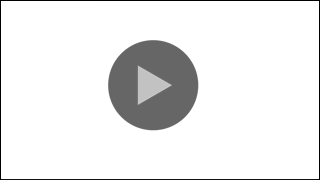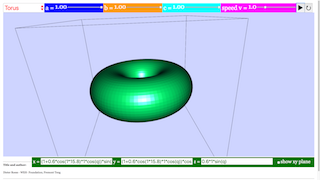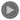Description

# 3D function plotter

## x = fx( p, q ); y = fy( p, q ); z = fz( p, q)

This function plotter displays animated 3D surfaces described by three coordinate functions of two variables p, q for the spatial coordinates x, y, z.

The coordinate functions fx , fy , fz , which in the simulation are shown in three editable text fields, map the points of the plane pq unambiguously into a surface in space xyz. If fx , fy ,, fz contain periodic functions of the parameters p and q, closed or intersecting surfaces may be produced.

The functions may contain four constants a, b, c, v that can be changed by sliders. In the predefined functions v is used to animate the surfaces by oscillating one or more of the coordinates via time dependent terms.

At the start of the simulation you will see the projection of a plane in space, viewed under perspective distortion. It is embedded into an x y z tripod, and is accompanied by the x y- plane z = 0. This plane can be deactivated by its check box.

Other predefined surfaces in space can by selected in the ComboBox.

The formula for the plane (pi ≡ π) shows that v modulates the z-function periodically: z = cos(vt)(a - 0.6)p. For t = 0 the modulation factor is 1; a determines the degree of modulation; (a- 0.6) defines a reasonable initial value. The other variable constants b, c are not used with the plane.

Play starts the animation, with time t starting at 0, as indicated in the t number field. With cos(t) fluctuating periodically, the plane oscillates in space. Slider a defines the base orientation. Pause freezes the animation at any spatial position. Reset leads back to the initial conditions.

The range of p and q is ∓ π . Scaling of all three axes x, y, z has a range of ∓ 1. The xy-plane cuts the z axis at the center of the z- arrow. As variables p and q change in the range to +π, a periodic function as cos(p) completes a full period in the variable plane.

The orientation of the tripod in space can be changed by drawing with the mouse.

Other ways of visualization are described in the next page.

Predefined functions are selected in the ComboBox with a mouse click. Constants a,b,c can be varied by sliders while the animation is running. By editing the formulas you can change the terms that are animated. You can input new parametric formulas to create your own surfaces. Do not forget to press the ENTER key after a change!

Touching a surface with the mouse pointer lets its color filling disappear; the wire mesh of calculation will be pronouncedly visible.

Visualization

# Visualization alternatives

Rotation:  Mark any point within the tripod by the mouse pointer and draw (while the left mouse key stays pressed).

Shift:  Draw while the Strg key is pressed.

Zoom:  Draw while the Shift key is pressed

Correction: To return to the default projection choose another surface, and then the oldone anew

Show coordinates:  Mark a point on the surface while pressing the Alt key. When you draw, a cutting plane will pass through the surface. Depending on orientation, different cuts can be evaluated.

Camera- Inspector: Press the right mouse key. A context menu will appear. Choose Elements option/ drawing 3D panel/ Camera. The Camera Inspector will appear. It will stay visible until it is deactivated. It offers the following options:

Perspective:  Distant lines appear shorter than near ones.

No perspective:  No perspective distortion.

Planar xy or yz or yx : One looks onto the respective planes.

Other options: Degree and angle of perspective can be defined.

Optimizing  parameters. The spatial impression can be optimized by adjusting parameters. The optimum will be different for different projections.

The context menu also offers programs for producing a picture or a video.

functions

Predefined functions

As p and scale in pi (π), for all terms where p and q enter directly into formulas for x ,y, z (e.g not in periodic function of the variables), a factor 1/pi (1/π) appears. A factor cos(v*t) indicates that the associated term is animated. Reset redefines t = 0 and hence cos(vt) = 1.

Fixed numbers in the formulas are used to define a reasonable scaling at the start of the simulation for uniform default values of parameters a, b, c (= 0.5).

x_function = p/pi

y_function = q/pi

z_function = cos(v*t)*(a/pi-0.6)*p

Plane

x_function = p/pi

y_function = q/pi

z_function = cos(v*t)*p*q/pi^2

x_function = cos(v*t)*a*cos(p)

y_function = b*sin(p)

z_function = c*q/(2*pi)

Cylinder

x_function = a*cos(p)*(1+q/(2*pi)*cos(p/2))

y_function = 2*b*sin(p)*(1+q/(2*pi)*cos(p/2))

z_function = c*q/(pi)*sin(p/2*t)

Möbius strip

x_function = cos(v*t)*a*cos(p)*abs(cos(q))

y_function = cos(v*t)*a*sin(p)*abs(cos(q))

z_function = cos(v*t)*a*sin(q)

Sphere

x_function = a*cos(p)*abs(cos(q))

y_function = cos(v*t)*b*sin(p)*abs(cos(q))

z_function = c*sin(q)

Ellipsoid

x_functio n= a/pi*q*cos(p)*cos(v*t)");

y_function = b/pi*q*sin(p)*cos(v*t)");

z_function = c*q/pi");

Double cone

x_function = (a+0.6*cos(v*t)*b*cos(q))*sin(p)

y_function = (c+0.6*cos(v*t)*b*cos(q))*cos(p)

z_function = 0.6*b*sin(q)

Torus

x_function = 2*(a+0.3*b*cos(q))*sin(p)*cos(p)

y_function = 2*((cos(v*t)^2)*c+0.3*b*cos(q))*cos(p)*cos(p)*cos(p)

z_function = 0.6*b*sin(q)

Torus-8

x_function = (cos(v*t)*c+0.3*b*cos(q))*cos(p)*cos(p)*cos(p)

y_function = (a+0.3*b*cos(q))*sin(p)

z_function = b*0.3*sin(q)

Mouth

x_function = (0.4*c+0.4*b*cos(q))*cos(p)*cos(p)*cos(p)

y_function = (2*a+0.4*b*cos(q))*sin(p)

z_function = cos(v*t)*0.4*b*cos(q)

Boat_1

x_function = (0.4*c+0.4*b*cos(q))*cos(p)*cos(p)*cos(p)

y_function = (2*a+0.4*b*cos(q))*sin(p)

z_function = cos(v*t)*0.4*b*cos(q)*cos(q)

Boat_2

experiments

E1: Test the different surfaces without a change of parameters. Rotate the frames and train your 3D perception of these functions.

E2: Study the formulas and develop a sense for the relation between formulas and surface.

E3: Rotate, tilt and zoom the 3D projection to optimize the visual impression of the surface . Use the Camera Inspector, too (see Visualization page).

E4: Vary parameters and study the influence on the appearance of the surface.

E5: Change signs (+/-) in the formulas and study the effect.

E6: Vary the grade of power functions in the formulas and study the resulting surfaces.

E7: Edit the formulas freehandedly and consider in advance how that should influence the surface.

E8: Animate different parameters, or two at the same time.

E9: Delete the animation term and use p as a free fourth parameter in your own formula.

E10: Reflect in using 3D surfaces created this way in design of 3D objects. The last three predefined functions are intended as encouragement (mouth and boat). Remember that numerical machines could directly use the formulas for control.

### Translations

Code Language Translator Run### Software Requirements

SoftwareRequirements

 Android iOS Windows MacOS with best with Chrome Chrome Chrome Chrome support full-screen? Yes. Chrome/Opera No. Firefox/ Samsung Internet Not yet Yes Yes cannot work on some mobile browser that don't understand JavaScript such as..... cannot work on Internet Explorer 9 and below

### CreditsDieter Roess - WEH- Foundation; Fremont Teng; Loo Kang Wee

[text]

## Parametric Surface 3D Simulator JavaScript Simulation Applet HTML5

### Instructions

#### Object Combo Box

Toggling through the combo box will give the various base shapes selected.

(Plane Selected)

(Cylinder Selected)

(Moebius Strip Selected)

(Spiral Selected)

(Sphere Selected)

(Ellipsoid Selected)

(Double Cones Selected)

(Torus Selected)

(Torus-8 Selected)

(Mouth Selected)

(Boat 1 Selected)

(Boat 2 Selected)

#### Sliders

Adjusting the sliders will modify their respective variables.

#### Analytical Surface Equation Boxes

Typing inside these boxes will change the shape of the curve as well,
and parse it as a new function.

#### Show xy-plane Check Box

Checking this will toggle the visibility of the cyan xy plane.

(Not Checked)

(When Checked)

#### Toggling Full Screen

Double click anywhere in the panel to toggle full screen.
Note that it won't work if the simulation is playing.

#### Play/Pause and Reset Buttons

Plays/Pauses and resets the simulation respectively.

Research

[text]

[text]

[text]

# Testimonials (0)

There are no testimonials available for viewing. Login to deploy the article and be the first to submit your review!